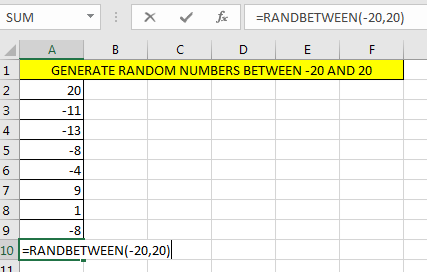# Excel RANDBETWEEN Function

The RANDBETWEEN returns a random whole number between specified range.RANDBETWEEN Syntax

=RANDBETWEEN(lower range, upper range)

RANDBETWEEN Example.

Lets say I want to get a random number or list of numbers, between -20 and 20.

Write this RANDBETWEEN formula in excel

=RANDBETWEEN(-20,20)

And you’ll have a random number between -20 and 20.

• RANDBETWEEN refreshes every time when something changes in workbook.
• RANDBETWEEN function is mostly used to randomize a list in Excel or generate random whole number

So, yeah you can use this function to random numbers between two ranges. Feel free to ask any questions about randomizing anything here.

Related Articles:

Get the Rank using SUMPRODUCT function

Count Unique Values using SUMPRODUCT function In Excel

2 Ways to Sum by Month in Excel

Count total matches using SUMPRODUCT in two ranges in Excel

Popular Articles:

How to use the VLOOKUP Function in Excel

How to use the COUNTIF function in Excel 2016

How to Use SUMIF Function in Excel

Terms and Conditions of use

The applications/code on this site are distributed as is and without warranties or liability. In no event shall the owner of the copyrights, or the authors of the applications/code be liable for any loss of profit, any problems or any damage resulting from the use or evaluation of the applications/code.